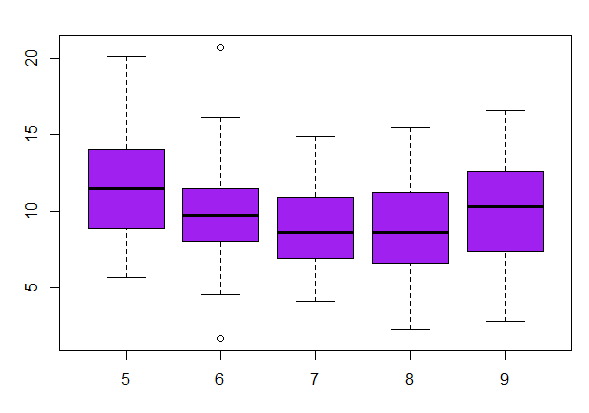[This article was first published on R Language in Datazar on Medium, and kindly contributed to R-bloggers]. (You can report issue about the content on this page here)
Want to share your content on R-bloggers? click here if you have a blog, or here if you don't.

The greatest value of a picture is when it forces us to notice what we never expected to see. — John W. Tukey. Exploratory Data Analysis.

### Why do we use exploratory graphs in data analysis?

• Understand data properties
• Find patterns in data
• Suggest modeling strategies
• “Debug” analyses

Data –We will use the air-quality dataset available in R for our analysis.The entire project can be found here. You can go and try it for yourself by running it on Datazar.

library(datasets)
head(airquality)

### Summaries of Data

One dimensional Data– Univariate EDA for a quantitative variable is a way to make preliminary assessments about the population distribution of the variable using the data of the observed sample.

When we are dealing with a single datapoint, let’s say temperature or, wind speed, or age, the following techniques are used for the initial exploratory data analysis.

• Five-number summary- This essantially provides information about the minimum value, 1st quartile, median, 3rd quartile and the maximum.
summary(airquality$Wind)Summary Of Windspeed • Boxplots– boxplot consists of a rectangular box bounded above and below by “hinges” that represent the quartiles Q3 and Q1 respectively, and with a horizontal “median” line through it. You can also see the upper and lower “whiskers”, and a point marking a potential “outlier”. IQR (interquartile range) = Q3 — Q1, (the box in the plot) whiskers = ±1.58IQR/√ n ∗ IQR, where n is the number of samples. (datapoints) boxplot(airquality$Wind~airquality$Month,col=”purple”)Wind Speed by Month • Histograms- The most basic graph is the histogram, which is a bar plot in which each bar represents the frequency (count) or proportion (count/total count) of cases for a range of values. Typically the bars run vertically with the count (or proportion) axis running vertically. To manually construct a histogram, define the range of data for each bar (called a bin), count how many cases fall in each bin, and draw the bars high enough to indicate the count. hist(airquality$Wind,col=”gold”)
rug(airquality$Wind)#(Optional)plots the point below in a histogram • Barplot- A bar chart is made up of columns or rows plotted on a graph. Here is how to read a bar chart made up of columns. • The columns are positioned over a label that represents a categorical variable . • The height of the column indicates the size of the group defined by the column label. • A bar chart is used for when you have categories of data: Types of movies, music genres, or dog breeds.Hence, a bar chart is used (and not histogram) when we are dealing with categorical variables. barplot(table(chickwts$feed),col = “wheat”, main=”Number Of Chickens by diet type”)

Two dimensional Data– Multivariate non-graphical EDA techniques generally show the relationship between two or more variables in the form of either cross-tabulation or statistics.

• Scatter Plot- This essantially provides information about the minimum value, 1st quartile, median, 3rd quartile and the maximum.

For two quantitative variables, the basic graphical EDA technique is the scatterplot which has one variable on the x-axis, one on the y-axis and a point for each case in your dataset. If one variable is explanatory and the other is outcome, it is a very, very strong convention to put the outcome on the y (vertical) axis.

One or two additional categorical variables can be accommodated on the scatterplot by encoding the additional information in the symbol type and/or color.

We will use the Males.csv dataset (present in the project on Datazar, to check whether being a part of an union impacts the salaries of young american males.

males<-read.csv(“dataset0.csv”)
samplemales<- males[1:100,] # we used first 100 rows
with(samplemales ,plot(exper,wage, col= union))
#union is a categorical variable represented by colorScatter plot to represent age vs experience (the color represent whether the employee is a part of an union)

We can also use multiple scatter plots to understand better, whether being part of an union impacts an employees salary.

We can see that, most employees are not part of an union and they tend to earn more than employees who are a part of an union.Correlation doesn’t always mean causation, as it might be the case, the high paying industries do not allow their employees to form unions.

In a nutshell: You should always perform appropriate EDA before further analysis of your data

Lastly, I wish you all a merry Christmas and a very happy new year. I will come back with the next edition of EDA in New Year. Till then, happy modeling!Exploratory Data Analysis Using R (Part-I) was originally published in Datazar on Medium, where people are continuing the conversation by highlighting and responding to this story.